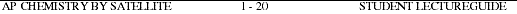###Go to Main Index

AP Chemistry by Satellite Lectureguide
Student Edition
Introduction to Chemistry: Matter and Measurement
Chapter 1

Objectives

Following your study of this chapter, you should be able to

1. define matter.
2. identify the important physical and chemical properties for some common substances.
3. describe identifying characteristics of the three states of matter.
4. differentiate between homogeneous and heterogeneous mixtures.
5. define the term atom, element and compound.
6. differentiate among elements, compounds and mixtures.
7. list postulates of Dalton's atomic theory.
8. visualize mixtures, pure substances, elements, compounds, gases, liquids and solids at the atomic level.
9. write the symbol for any of the first 36 elements in the periodic table, given the name.
10. write the name for any of the first 36 elements in the periodic table, given the symbol.
11. describe various separation techniques.
12. list the fundamental properties chosen as the basis for SI.
13. list the fundamental properties for derived units of measurement.
14. identify multiplier indicated by selected prefixes in the SI system and perform simple conversions.
15. distinguish between mass and weight.
16. calculate the density of a substance and perform calculations using density.
17. perform conversions between temperature scales.
18. distinguish between intensive and extensive properties.
19. distinguish between accuracy and precision.
20. distinguish between exact and inexact numbers.
21. determine the number of significant figures in a measured quantity.
22. determine the number of significant figures to report in the result of mathematical calculations.
23. use dimensional analysis to analyze problems.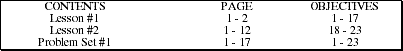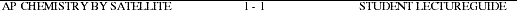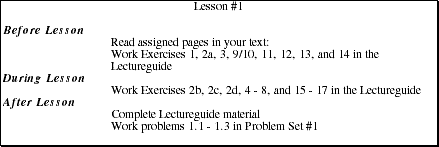1. List two necessary defining characteristics of matter and illustrate with examples.2a. List some physical properties for the substances below that were emphasized during the Lesson. (Note: You can use your text or the CRC Handbook of Chemistry and2a. (Continued)2b. List some physical properties for aluminum and bromine.2c. Describe the chemical change you observed when aluminum is added to bromine.2d. Explain why the reaction between aluminum and bromine does not occur immediately.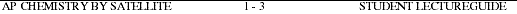3. Distinguish between the gas, liquid and solid phase by listing the unique properties of each that are not shared by the others.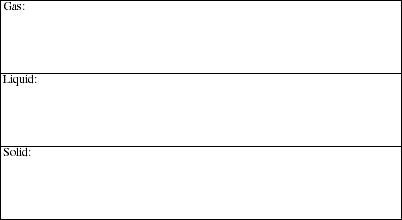4. Distinguish between pure substances, homogeneous mixtures and heterogeneous mixtures by listing the unique properties of each that are not shared by the others.5. Define the terms atom, element and compound (You may use drawings to support and clarify your definitions.)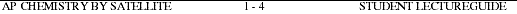6. Distinguish between element and compound by listing the unique properties of each that are not shared by the other.7. State the principle postulates of Dalton's atomic theory.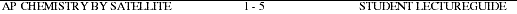8. Each diagram (A - P) show a sample of substances as viewed at the atomic level. Characterize the contents of the container in terms of each of the following categories:

Category I. Homogeneous mixture, heterogeneous mixture or pure substance Category II. Element(s), compound(s) or both
Category III. Solid, liquid, gas or combination of phases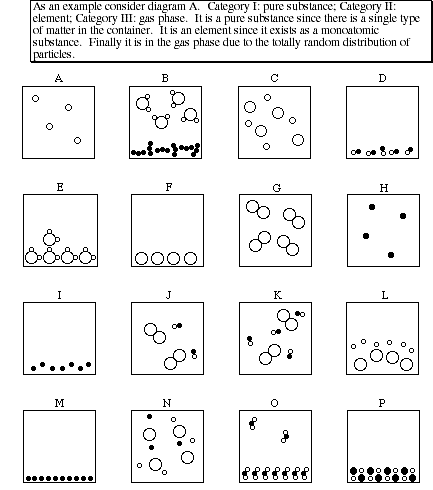8. (Continued)9/10. Write the names and symbols of the first 36 elements in the periodic table.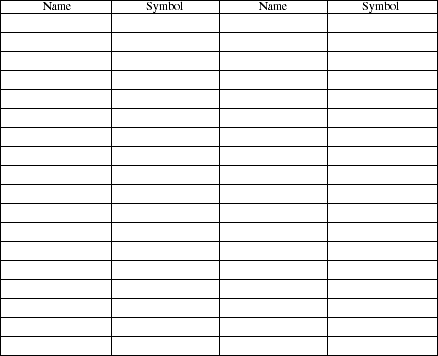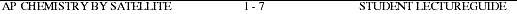11. For each of the separation techniques listed below, give an example of how the technique is used to separate the components of a mixture.

a) Decantationb) Filtrationc) Evaporationd) Distillatione) Paper chromatographyf) Liquid-solid chromatography12/13. Units for which of the following physical quantities are base units in SI and which are SI-derived units? For each provide the name and symbol (abbreviation) of the SI unit used to express physical quantity.14. Give the name of the prefix and its abbreviation for each of these multipliers.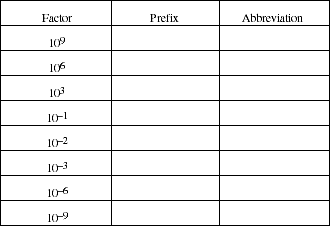15. Distinguish the meaning of the terms mass and weight.16. Figure I shows a glass cylinder containing four liquids each of different density. Two of the liquids have been identified. A table containing a list of substances and their density (at 25 ºC) has been provided. From the list select a substance for Liquid #1 and Liquid #3. Briefly explain the reason(s) for your selections and for the remaining substances the reason they were not selected.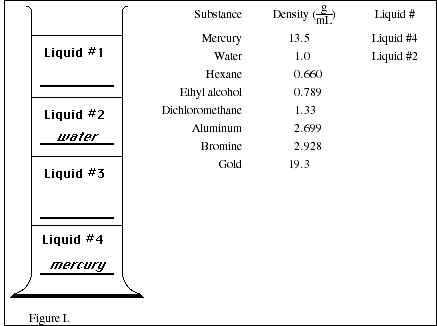17. The temperature of liquid ethanol was measured several times with two thermometers as the sample was heated from its freezing point to its boiling point. One thermometer was calibrated in degrees Celsius, the other in degrees Fahrenheit. The data collected is shown in the table below.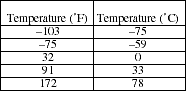a) Plot the data with ºF on the y-axis and ºC on the x-axis. Determine the mathematical equation relating ºF to ºC.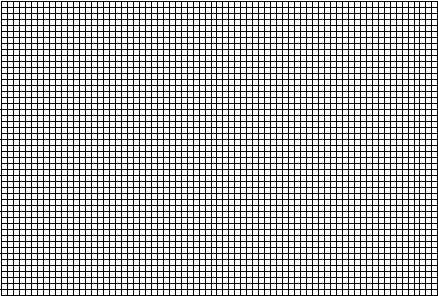b) Use the equation obtained from your graph to calculate the Fahrenheit temperature corresponding to -40 ºC.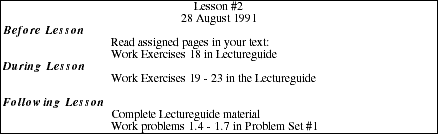18. Distinguish between extensive and intensive properties. Classify each of the physical properties listed as extensive or intensive.

Physical Property Classification

volume

mass

density

temperature

heat

length

time

19a. Compare and contrast accuracy and precision.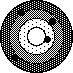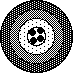ABCb) Which set(s) of measurements are precise?

c) Which set(s) of measurements are accurate?

d) Which set(s) of measurements are both precise and accurate?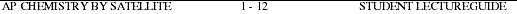20a. Explain the difference between an exact number and an inexact number.b) For each case, indicate whether the result is an exact or inexact number.

(i) The number of apples: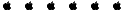(ii) The mass of six apples

(iii) The number of seeds in the apples in (i)

(iv) The number of feet in one mile

(v) The number of liters in five quarts

21. List the procedures for determining the number of significant figures in a measured quantity. (Be particularly careful to distinguish between zero as a place-holder and zero as a significant figure.)22a. List the procedures for determining significant figures in the result of calculations involving measured quantities.22b. List the rules for rounding off numbers.c) Complete the following calculations and record the result using the correct number of significant figures.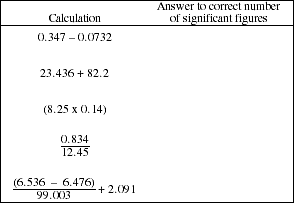23a. List some important unit conversion factors.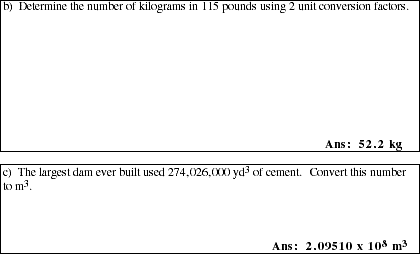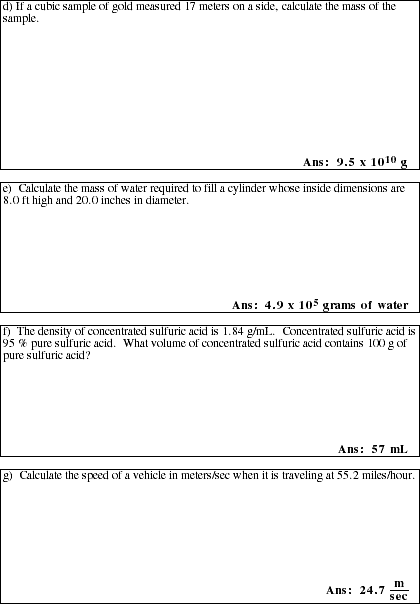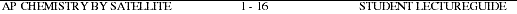AP Chemistry by Satellite
Problem Set #1

ALL work must be shown to receive full credit.

PS1.1. Diagram each of the following systems as viewed at the atomic level in the space provided.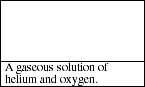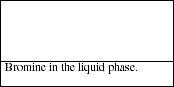PS1.2. A golden colored cube measures 2.00 cm on each side and has a mass of 154.4 g. Could the cube be made of gold? Explain your answer using both words and a mathematical calculation.PS1.3. Which temperature of each pair is lower: (Justify each answer with a calculation.)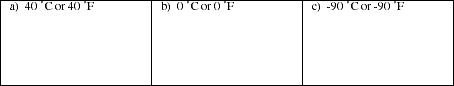PS1.4. The approximate volume of water contained in the oceans of the world is 3.3 x 108 mi3. Determine the volume in km3.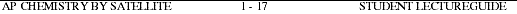PS1.5. A line of water molecules 2.5 inches long contains 2.5 x 108 molecules. Calculate the diameter of a water molecule in centimeters, angstroms and nanometers.PS1.6. The density of water is 1.00 g/mL. What is the weight, in pounds of 1.00 gallon of water?PS1.7a During a flood an average of 1.00 x 103 ft3 of water flowed out of a dam's flood gate each minute. What volume in ft3 and liters flowed out of the gate in one hour?b) If the flow was constant for one entire day, what mass of water in kilograms was released from the dam? What weight in pounds?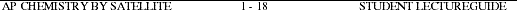PS1.8. Using a standard linear measuring device (ruler) measure the length and width of this sheet of paper in both inches and centimeters. (Be sure to report the appropriate number of significant figures). Find the area of this page in square centimeters.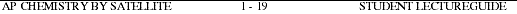Microcomputer software

Project SERAPHIM
Dr. John Moore
Department of Chemistry
University of Wisconsin

AP102 SIGNIFICANT FIGURE DRILL
GRAPH

AP104 ACCURACY AND PRECISION OF LAB MEASUREMENTS DIMENSIONAL ANALYSIS (requires 80-column card)Introduction to General Chemistry by Stan Smith, Ruth Chabay and Elizabeth Kean Drill-and-practice software
\$500 (10-disk set)

Falcon Software
P.O Box 200
Wentworth, NH 03282
1-603-764-5788

Diskette #10 The Metric SystemComputer Aided Instruction for General Chemistry by William Butler & Raymond Hough Drill-and-practice software
\$40 (4-disk set)

John Wiley & Sons, Inc.
605 3rd Avenue
New York, NY 10158
(this software may not be available)

Diskette #1 Introduction: Units and Measurement